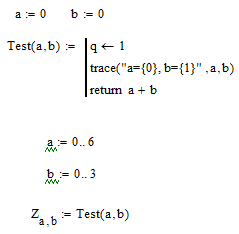cancel
Showing results for
Did you mean:
cancel
Showing results for
Did you mean:

## Nested Range Variables order of evaluation4-Participant

## Nested Range Variables order of evaluation

If you have nested range variables, which loop gets executed fastest?

For example in the following the trace indicates the inner loop gets executed fastest, but if you swap the a and b lines, the outer loop loop now seems to be executed fastest???6 REPLIES 624-Ruby V
(To:ptc-2060417)

In this crappy forum you unfortunately can't just copy and paste pictures to your posts. To embed pictures you have to use the camera icon from the menu (which meas you have to save the picture to a file first)

Its not clear to me how you would time execution time by looking at the traces!?

Its also not clear what exactly you mean with  "swap the a and b lines" and which "loop" you would consider the "outer" one.

Do you think that one of the calculations below executes faster than the others?
Z4 and Z5 should be a little bit faster, I guess.BTW, the above calculations can be speeded up significantly by allocating space for the array before the loops4-Participant
(To:Werner_E)

I cut & pasted the formula from Mathcad into the post and it showed it correctly, but when it showed me the review it was converted to an icon. Oh well...

I'm not trying to measure execution time here.

In the attached file in my post I consider the "b" loop as the inner one and the "a" loop as the outer. If you run this you get a trace like this:

a=0, b=0
a=0, b=1
a=0, b=2
a=0, b=3
a=1, b=0
a=1, b=1
a=1, b=2
a=1, b=3
a=2, b=0 .........

which indicates to me that the outer loop "a" is set to 0 and then the inner loop "b" is run from 0 to 3, then "a" is set to 1 and "b" again run from 0 to 3 and so on........

If you then swap the range lines i.e. change

a:=0..6

b:=0..3

to

b:=0..3

a:=0..6

then the trace print out does not change. This indicates to me that now the inner loop variable is set to 0 and the outer is run from 0 to 3, then then inner set to 1 and the outer run again from 0 to 3.

I expected that in every case the outer would be set to 0 and the inner run over its range, then the outer set to 1 and the inner run over its range again etc...  But it appears to be inconsistent in this.

So my question is how does Mathcad decide which loop it will hold constant while the other is run over its range??

Roger24-Ruby V
(To:ptc-2060417)

You wrote "now seems to be executed fastest" and so I guessed you were talking about execution time.

So you really think that the order the two ranges a and b are defined has any influence on the way Mathcad loops over Z[a,b ? Why do you think so? Changing the order of the definitions of a and b does not has any effect on later calculations which use a and b.

The expression Z[a,b stands on its own when it comes to execution - when it sees the indices a and b it looks up their value and it does not matter at all where on the sheet those variables were defined (as long as its above or to the left). Z[a,b always evaluates with a as the outer "loop" simply because its the index placed first (row index).

Z[b,a evaluates the other way round and will of course give you the transposed matrix.4-Participant
(To:Werner_E)

I understand that inside loops Mathcad will execute each line over the loop ranges and then move on to the next line.

My question is when executing a line inside more than 1 loop, how does it decide which order to evaluate the loop ranges that apply to that line?

R23-Emerald I
(To:ptc-2060417)

The loops execute in the order written; if you have

i = 0..3

j = 0..6

The i will be set to 0  and j run thru its range, then i will be increnemted  and j run thru its range again, . . .

The print out doesn't change because the subscript designates rows and columns:

A[i,j means that the value of i will determine which row and the value of j will determine which column is filled.

Werner's example showed the output changing shape (rows vs columns).24-Ruby V
(To:Fred_Kohlhepp)

@Fred_Kohlhepp wrote:

The loops execute in the order written; if you have

i = 0..3

j = 0..6

This applies only to for-loops in a program. In case of the example ptc-2060417 provided the order the two ranges are defined does not matter at all. In R[i,j there are always the rows (i) executed outside and for every row the columns (j) are cycling one after the other. This is best seen with his traces in the debug window. There is no change no matter in what order the ranges are defined in the sheet.

The problem is that ptc-2060417 is just defining range variables but is always talking about "loops".

While its correct to say that a range is something like an implicit loop, I really don't understand what he means when he writes

"My question is when executing a line inside more than 1 loop, how does it decide which order to evaluate the loop ranges that apply to that line?"

ptc-2060417, you'll have to provide a more specific example of what you would like to know. What do you mean by "inside a loop" when you are not talking about real loops in a program but just mean range variables?